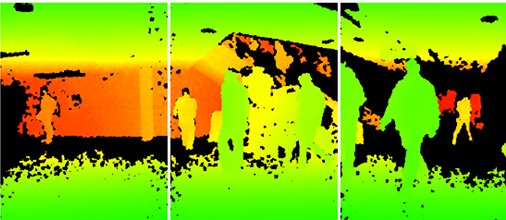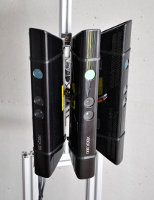# RGB-D People Dataset

###### Annotated people and tracks in RGB-D Kinect dataDescription

This dataset contains 3000+ RGB-D frames acquired in a university hall from three vertically mounted Kinect sensors. The data contains mostly upright walking and standing persons seen from different orientations and with different levels of occlusions.
This dataset has been re-annotated with respect to  and it contains minor annotation differences.

CLARIFICATION: Results in paper  are computed each three frames. Results in paper  are computed in each frame.

CLARIFICATION 2: Some people experienced problems in loading the depth images. In case that depth values are over 10^4, then swap the position of the two bytes for each depth pixel. It is a big/little endian interpretation problem.

Citations

If you are considering using this data, please reference the following:
•  L. Spinello, K. O. Arras. People Detection in RGB-D Data. IEEE Int. Conf. on Intelligent Robots and Systems (IROS), 2011.
•  M. Luber and L. Spinello and K. O. Arras People Tracking in RGB-D Data With On-line Boosted Target Models. IEEE Int. Conf. on Intelligent Robots and Systems (IROS), 2011.

The dataset can be download from http://www.informatik.uni-freiburg.de/~spinello/sw/rgbd_people_unihall.tar.gz

Sensory Setup

Three vertically mounted kinect have been assembled on a sensory tower at ~1.50m height. The setup has been chosen to minimize the IR projector cross-talk between the sensors. Data has been acquired @ 30Hz.Annotation Format

Each annotated track is defined in a file named as TRACK_000N.DAT . The file describes the position of the target in each depth frame it appears. The target visibility status is also annotated. The track-ID is encoded in the file name (TRACK_000N.DAT -> track-ID N). The content of a track file is as following:
`IMAGE_NAME_ID TS X_tl_dpt Y_tl_dpt WDT_dpt HGT_dpt X_tl_rgb Y_tl_rgb WDT_rgb HGT_rgb VSB  ... `
where:
```IMAGE_NAME_ID = image filename, seq0_0001_2.pgm [ID: camera id, 0 = left, 1 = center, 2 = right]
TS = timestamp
X_tl_dpt, Y_tl_dpt = topleft coord. bounding box in the depth image
WDT_dpt, HGT_dpt = size of the bounding box in the depth image
X_tl_rgb, Y_tl_rgb = topleft coord. bounding box in the RGB image
WDT_rgb, HGT_rgb = size of the bounding box in the RGB image
VSB = visibility in the depth image [0 = hidden  1  = fully visible, 2 = partially visible ]
TS = timestamp ```
Visual explaination:Depth images are saved as 16bits, 1 channel PGM images [640x480]. They contain the raw data content from the Kinect sensor. Namely, each pixel has value between [0,1084]. Please check this page in case you are interested in metric values.

Some people experienced problems in loading the depth images. In case that depth values are over 10^4, then swap the position of the two bytes for each depth pixel. It is a big/little endian interpretation problem.

RGB images are saved as 8bits, 3 channels PPM images [640x480].

Calibration

RGB intrinsics params:
```k1=2.6451622333009589e-01  k2=-8.3990749424620825e-01 k3=9.1192465078713847e-01
p1=-1.9922302173693159e-03 p2=1.4371995932897616e-03
fx=5.2921508098293293e+02 fy=5.2556393630057437e+02
cx=3.2894272028759258e+02 cy=2.6748068171871557e+02 ```
Depth intrinsics params:
```k1=-2.6386489753128833e-01  k2=9.9966832163729757e-01 k3=-1.3053628089976321e+00
p1=-7.6275862143610667e-04 p2=5.0350940090814270e-03
fx=5.9421434211923247e+02 fy=5.9104053696870778e+02
cx=3.3930780975300314e+02 cy=2.4273913761751615e+02 ```
Extrinsic params:
```T= [1.9985242312092553e-02  -7.4423738761617583e-04 -1.0916736334336222e-02]
{rotation angles} roll=1.22775236635173e-02 pitch=-1.74713102421449e-02 yaw=-1.47813574129019e-03
```